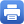Columbia Home
Modern Geometry

This course is taken in sequence, part 1 in the fall, and part 2 in the spring.

### Modern Geometry I

I Differential Manifolds

• Smooth manifolds
• Tangent spaces, tangent bundles
• Smooth maps (submersions, immersions, embeddings)
• Vector fields (flows, Frobenius’s theorem)
• Lie groups and homogeneous spaces
• Tensors, differential forms, Stokes’s theorem

II Riemannian Geometry

• Riemannian metrics and connections
• Geodesics
• Curvature
• Jacobi fields
• Isometric immersions (second fundamental form)
• Manifolds of constant curvature
• Bonnet-Myers theorem

### Modern Geometry II

I Differential Topology

• Transversality
• Tubular neighborhoods
• Intersection theory (mod 2 and oriented)
• Degrees
• Poincare-Hopf index theorem
• Lefschetz fixed-point theorem
• de Rham cohomology
• Poincare duality

II Vector Bundles and Principal Bundles

• Real and complex vector bundles
• Metrics, connections, and curvature on vector bundles
• Chern, Pontryagin, and Euler classes
• Principal bundles
• Connections and curvature on principal bundles
• Parallel transport and holonomyPrint this page